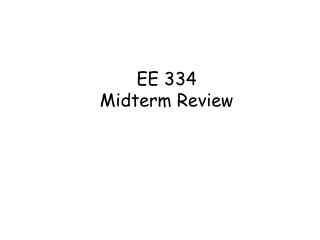# EE 334 Midterm Review - PowerPoint PPT PresentationDownload PresentationEE 334 Midterm Review

EE 334 Midterm ReviewDownload Presentation## EE 334 Midterm Review

- - - - - - - - - - - - - - - - - - - - - - - - - - - E N D - - - - - - - - - - - - - - - - - - - - - - - - - - -
##### Presentation Transcript

1. EE 334Midterm Review

2. Diode: Why we need to understand diode? • The base emitter junction of the BJT behaves as a forward bias diode in amplifying applications. • The behavior of the diode when reverse bias is the key to the fabrication of the integrated circuits. • The diode is used in many important nonamplifer applications.

3. Departure from ideal behavior • The four major reason why the actual diode do not correspond exactly to the ideal. • Ohmic resistance and contact resistance in series with the diode cause the VI • curve to become linear at high forward current. • Avalanche or Zener breakdown take place at high reverse voltage, causing an • abrupt increase in reverse current. • 3. Surface contaminants cause an ohmic layer to form across the junction, which is • Increasing the reverse current as reverse voltage is increased. • 4. Recombination of current carrier in the depletion region take place due to traps.

4. The purpose of modeling • Nonlinear problems are much more difficult than linear ones. These problems could be impossible to solve manually and could require huge amount of time if solved on a computer. • One possible solution of the above mentioned problem is to approximate the nonlinear relationship with a model that has a linear relationship. • The trust of nonlinear modeling is direct towards this end. • The modeling not only simplifies the solution, it also allows the designer to understand how the circuit behaves. Modeling often increases the conceptual understanding of the circuit operation.

5. Schottky Barrier Diode One semiconductor region of the pn junction diode can be replaced by a non-ohmic rectifying metal contact.A Schottky contact is easily formed on n-type silicon. The metal region becomes the anode. An n+ region is added to ensure that the cathode contact is ohmic. Schottky diodes turn on at a lower voltage than pn junction diodes and have significantly reduced internal charge storage under forward bias.

6. Reverse Breakdown Increased reverse bias eventually results in the diode entering the breakdown region, resulting in a sharp increase in the diode current. The voltage at which this occurs is the breakdown voltage, VZ. 2 V < VZ< 2000 V

7. Half WaveRectification

8. Figure 2.7 A full-wave bridge rectifier: (a) circuit showing the current direction for a positive input cycle, (b) current direction for a negative input cycle, and (c) input and output voltage waveforms

9. Half-wave rectifier with filter

10. Voltage regulation is the measure of circuit’s ability to maintained a constant output even when input voltage or load current varies. % regulation is used to measure how well the regulator is Performing its function.

11. Demonstration of Zener diode as a voltage regulator

12. The large value of VCE decreases the effective base width W. Since IS is inversely propositional to W, which cause increase in IC.

13. Bipolar NOR logic gate Example 3.11 Determine current and voltage in the circuit 3.43(b) Rc=1K RB=20K VBE(on)=0.7V VCE(sat)=0.2V β=50 Lecture #3

14. The process by which the quiescent output voltage is caused to fall somewhere the cutoff and saturated values is referred to as biasing.

15. Example 3.13

16. Q-point has shifted Substantially. Q-point is not stabilized Against the variation .

17. Chapter 4Small-Signal Modeling and Linear Amplification

18. DC and AC Analysis • DC analysis: • Find dc equivalent circuit by replacing all capacitors by open circuits and inductors by short circuits. • Find Q-point from dc equivalent circuit by using appropriate large-signal transistor model. • AC analysis: • Find ac equivalent circuit by replacing all capacitors by short circuits, inductors by open circuits, dc voltage sources by ground connections and dc current sources by open circuits. • Replace transistor by small-signal model • Use small-signal ac equivalent to analyze ac characteristics of amplifier. • Combine end results of dc and ac analysis to yield total voltages and currents in the network.

19. DC Equivalent for BJT Amplifier • All capacitors in original amplifier circuits are replaced by open circuits, disconnecting vI, RI, and R3 from circuit.

20. AC Equivalent for BJT Amplifier • Find ac equivalent circuit by replacing all capacitors by short circuits,

21. Hybrid parameter I: diffusion resistance/input impedance • The diffusion resistance r is define as the reciprocal of the iB-vBE curve, which can be find as,

22. Output terminal characteristics of the bipolar transistor: transconductance • If we assume constant collector-emitter voltage the, As we know

23. By using the two hybrid parameters (r, gm), we can develop a simplified small signal hybrid-- equivalent circuit for the npn transistor. • Voltage -controlled current source gmvbe can be transformed into current-controlled current source,

24. From equivalent circuit we can write as Voltage gain

25. If we include the early effect then collector current in terms of early voltage as, then

26. Summary of hybrid--model parameters Diffusion resistance Current gain transconductance Output resistance